# Divide

In this mini-lesson, we will explore the world of division.

You will get to learn about the interesting concepts related to the definition of division, the division symbol, the general formula of division, and the basic parameters of division.

Meera's teacher wants to divide a group of 8 children into four groups. She does this using division. You too can divide large groups by visualising division. Watch this video to know more.

What comes into mind when you hear the word "divide." Is it a division of cookies into equal parts among you and your siblings?

Let's consider an example.

Virat, Hardik, Raghav, and Aryan are arguing about dividing some cookies, muffins, and donuts between them equally.

Look at the pictures below and check whether the division has been done equally or not.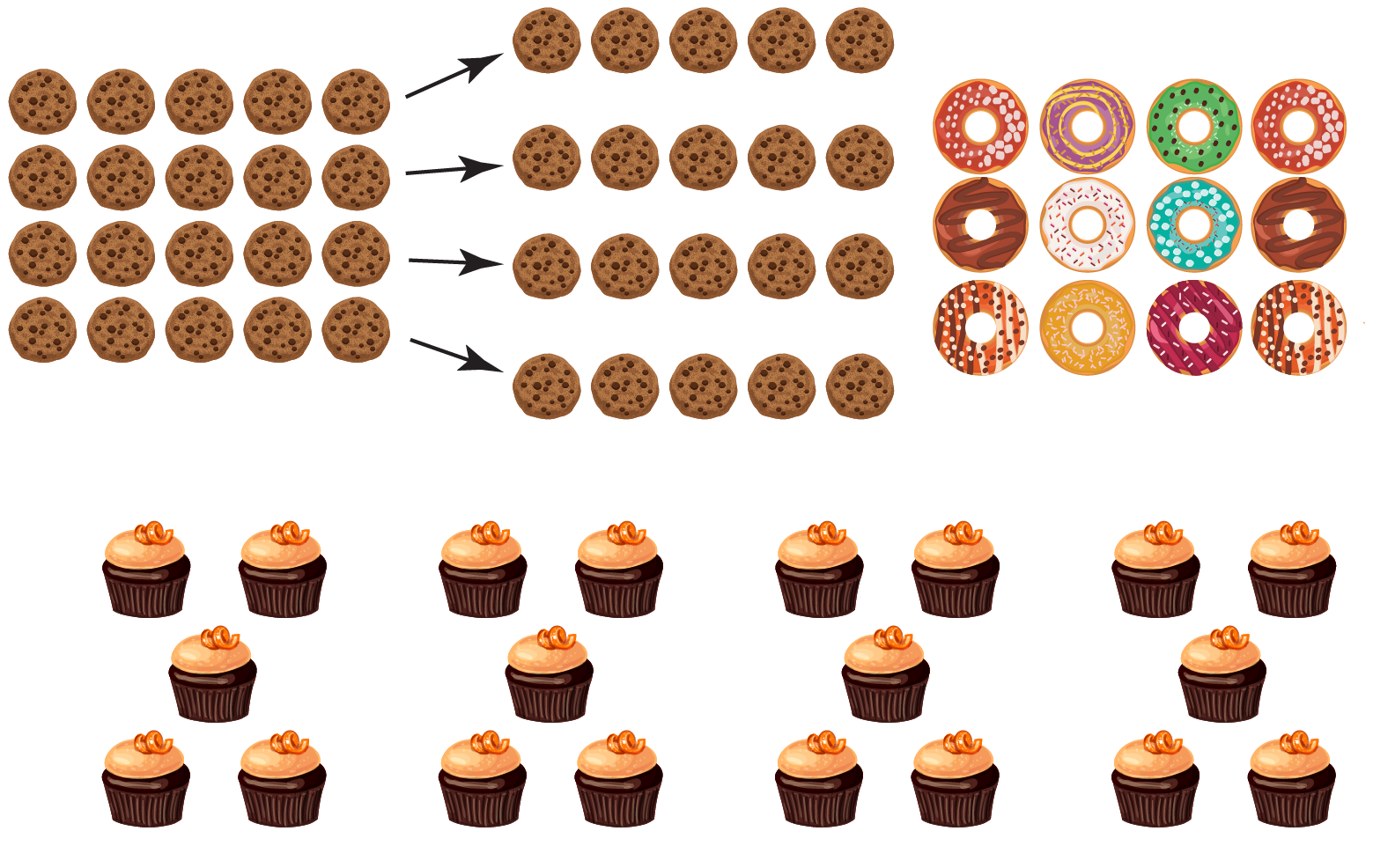The above image shows that all the snacks have been divided equally between them.

## Lesson Plan

 1 What is Division? 2 Solved Examples on Division 3 Interactive Questions on Division

## What is Division?

### Divide Definition

The division is a major arithmetic operation in which numbers are combined and divided in such a way that it forms a new number.

There are two basic divide symbols that represent division.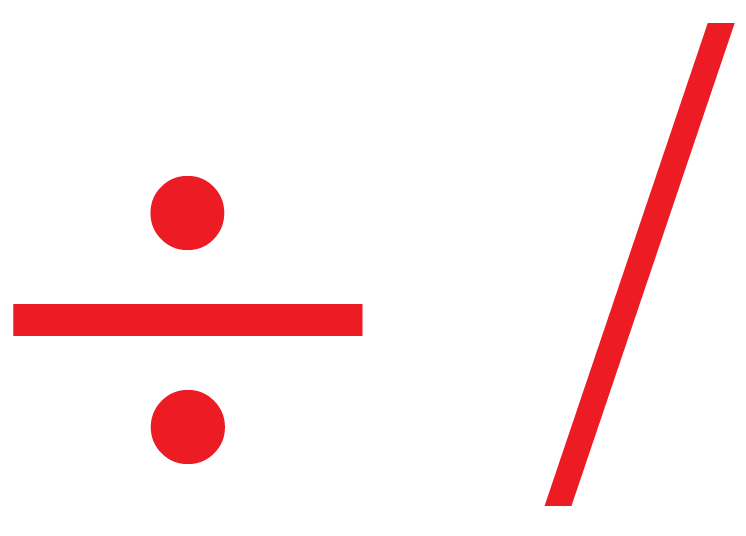The divide symbols are used in division statements.

The usage of divide symbol is shown below:

\begin{align} 4\div 2 &=2\\\\ 4 / 2 &= 2\end{align}

## What is the General Formula for Division?

The basic formula for division is given as:

 \begin{align} Dividend=Divisor\times Quotient+Remainder\end{align}

Look at the example below describing the various parameters of division.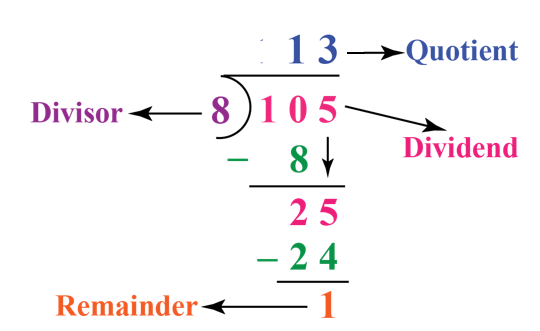### Division Calculator

Let us have some fun with math.

Move the sliders for dividend and divisor in the divide calculator shown below. Press start to observe the result.

Click reset for new observations.

You can observe how two numbers are divided and how the remainder is obtained.Important Notes

You can check the answers for division by using the following formula:

$$Dividend$$ = $$Divisor\times Quotient + Remainder$$

## Solved Examples

Let us look at some solved examples on division.

 Example 1

Lalit has 2 puppies.

He bought 8 chewable bones to feed them both equally.

How many bones will each puppy get?

Solution

$$\text{Number of Puppies} = 2$$
$$\text {Number of bones} =8$$
$$\text{Number of bones for each puppy} = 8\div 2$$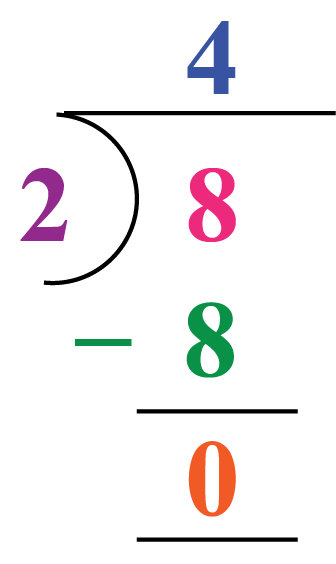\begin{align}\therefore\text{No. of bones for one puppy} &= 8\div 2 = 4\end{align}
 Example 2

Riya wants to celebrate her 6th birthday.

She bought 36 chocolates and wants to distribute them among her 6 friends.

How many chocolates will each of her friends get?

Solution

$$\text{Total friends}=6$$
$$\text{Total chocolates}=36$$
$$\text{No. of chocolates for each friend}=36\div 6$$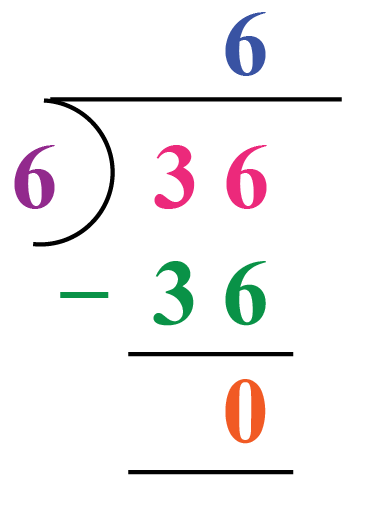\begin{align}\therefore \text{Chocolates for each friend} &= 36\div 6 = 6\end{align}
 Example 3

Aman's mother baked some cookies for him.

Priya and Aarav, his best friends, decided to give him a surprise by visiting him unannounced.

If there were 9 cookies, how many did Aman's mother give Aman, Priya, and Aarav so that they were equally shared between them?

Solution

$$\text{Number of cookies} = 9$$

$$\text{Cookies divided equally among Aman, Priya and Aarav}= 9\div 3$$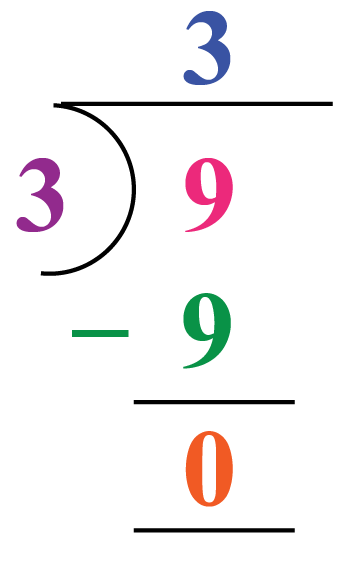To check division, we will put the values in the formula, \begin{align}Dividend &= Divisor\times Quotient \ + Remainder\\\ 9 &= 3\times 3 \ + 0\\ 9& = 9 \end{align}

 \begin{align}\therefore \text{Aman, Priya and Aarav got 3 cookies each}\end{align}Challenging Questions
1. Divide and check your answer for the following: (a) 85 ÷ 5 (b) 666 ÷ 6
2. Find the quotient and remainder: (a) 655 ÷ 10 (b) 203 ÷ 100
3. Write down a division fact for each of the following multiplication examples:
(a) 9 × 4 = 36
(b) 6 × 5 = 30

## Interactive Questions

Here are a few activities for you to practice. Select/Type your answer and click the "Check Answer" button to see the result.

## Let's Summarize

We hope you enjoyed learning about Divide with the simulations and practice questions. Now, you will be able to easily solve problems on the divide symbol, use the divide calculator, and recall the divide definition in math.

At Cuemath, our team of math experts is dedicated to making learning fun for our favorite readers, the students!

Through an interactive and engaging learning-teaching-learning approach, the teachers explore all angles of a topic.

Be it worksheets, online classes, doubt sessions, or any other form of relation, it’s the logical thinking and smart learning approach that we, at Cuemath, believe in.

## 1)What are the two types of division?

The division is split into two parts i.e., partitive and quotative models.

Partitive is used when dividing a number into a known number of slots. For example, if we divide 4 into 2 slots, we can find out how many items will be in each slot.

Quotative division is used when dividing a number into slots of a measured quantity. For example, when we divide 4 into slots of 2, we can determine how many slots can be created.

## 2)How do you divide one digit by another digit?

While dividing one digit by another digit, we can directly use the multiple (multiplication table) of the particular number.

For example, $$\ 9 \div 3 = 3$$  or $$\ 3\times 3 = 9$$

## 3)How do you explain dividing?

The division is a process of segregating two or more things.

Mathematically, division is an arithmetic operation used to form a new number from a combination of different numbers.

For Example, $$\ 9 \div 3 = 3$$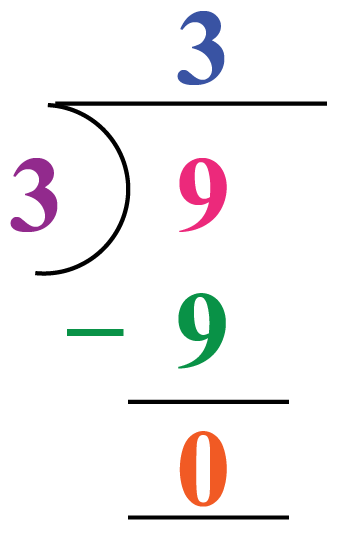More Important Topics
Numbers
Algebra
Geometry
Measurement
Money
Data
Trigonometry
Calculus